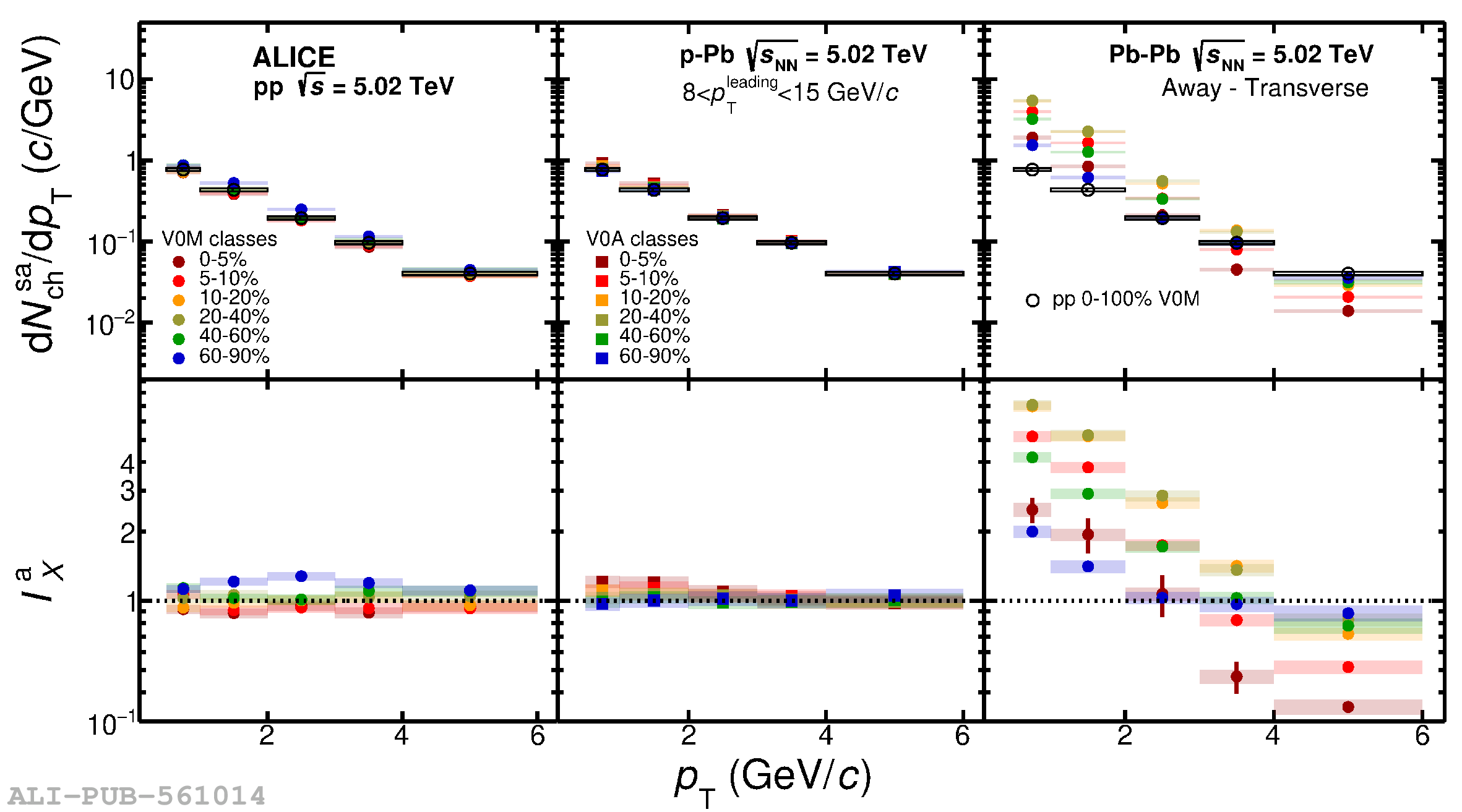# Figure 3

 \pt spectra of charged particles in Toward-Transverse, ${\rm{d}}N_{\rm{ch}}^{\rm{st}}/{\rm{d}}p_{\rm T}$ (top plot) and Away-Transverse, ${\rm{d}}N_{\rm{ch}}^{\rm{sa}}/{\rm{d}}p_{\rm T}$ (bottom plot) regions for different multiplicity classes in \pp (left), \pPb (middle) and \PbPb (right) collisions at $\sqrt{s_{\rm NN}}=5.02$\,\TeV. The lower panels of both plots show the ratio to minimum bias \pp collisions. The statistical and systematic uncertainties are shown by bars and boxes, respectively.